# Finding factors of a number | Factors and multiples | Pre-Algebra | Khan Academy

##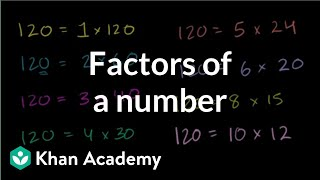By Khan Academy

To find the factors of a number means to find all the whole numbers that the number in question is divisible by. Can you help us find the factors in this example?# How to find all the factors of a given number - 4th grade math

##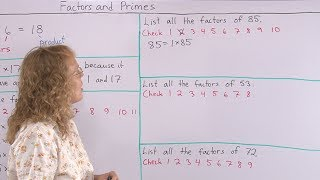By MathMamoth

I explain a systematic way of finding all the factors of a given number.# Finding Factors of a Number

##By Khan Academy

To find the factors of a number means to find all the whole numbers that the number in question is divisible by. Can you help us find the factors in this example?# Finding Factors of a Number

##By Khan,Academy

To find the factors of a number means to find all the whole numbers that the number in question is divisible by. Can you help us find the factors in this example?# Prime Factors of a Number | MathHelp.com

##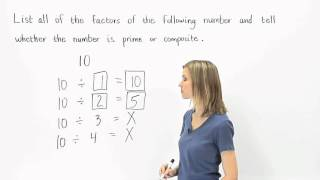By MathHelp.com# Prime numbers | Factors and multiples | Pre-Algebra | Khan Academy

##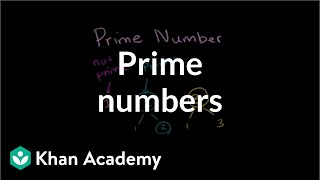By Khan Academy

What does it mean to be a prime number? Let's progress though some whole numbers and ask ourselves if they meet the criteria. What is the criteria you ask? Watch.# Recognizing prime and composite numbers | Factors and multiples | Pre-Algebra | Khan Academy

##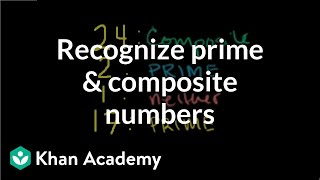By Khan Academy

Can you recognize the prime numbers in this group of numbers? Which are prime, composite, or neither?# Prime Factorization

##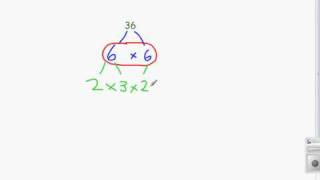By TeacherTube Math

WEBSITE: http://www.teachertube.com Finding the prime factorization of a number using factor trees# Factor pairs

##By Khan Academy

To find the factors of a number means to find all the whole numbers that the number in question is divisible by. Can you help us find the factors in this example?# Identifying factors and multiples

##By Khan Academy

To find the factors of a number means to find all the whole numbers that the number in question is divisible by. Can you help us find the factors in this example?# Finding LCM by Listing Factors

##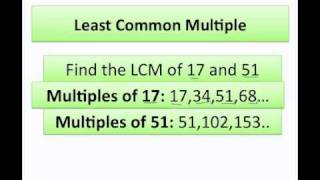By Vanessa Graulich

Learn to find LCM of numbers by listing their factors.# Factorization and prime numbers

##By MathPlanetVideos

Factorize the expression 12x^3y^2# Finding Factor Pairs

##By Skubes ed

Find all factor pairs for a whole number in the range 1–100.# [4.OA.4-2.0] Factor Pairs less than 100 - Common Core Standard

##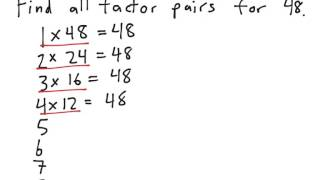By Freckle education

Find all factor pairs for a whole number in the range 1–100.# Khan Academy: Greatest Common Divisor

##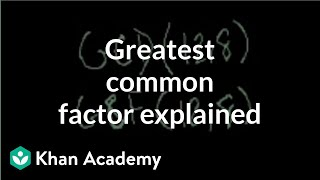By Khan Academy

Watch this video on finding the greatest common divisor or greatest common factor of two numbers. The instructor clearly explains the process and shows the steps for finding the greatest common factor of two numbers.# Equivalent forms of expressions with complex numbers

##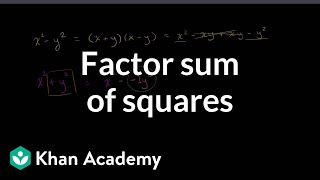By Khan Academy

Learn how expressions of the form x^2+y^2 can be factored into linear factors. This would not be possible without the aid of complex numbers!# Greatest Common Factor | GCF | MathHelp.com

##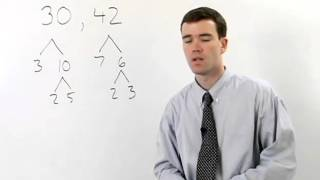By MathHelp.com# Division as an Unknown Factor

##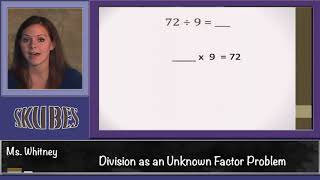By Skubes ed

Understand division as an unknown-factor problem. For example, find 32 ÷ 8 by finding the number that makes 32 when multiplied by 8.# Factoring Fun (4.OA.B.4)

##By Madison`s Math

fourth graders are expected to find all factor pairs for the numbers 1 through 100.# Using upside down birthday cake to find the greatest common factor (GCF)

##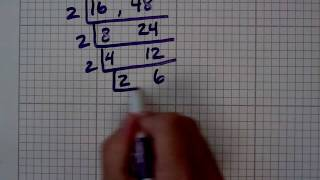By Nancy Foote

This is an alternate way to determine the greatest common factor (GCF) for a set of numbers using the upside down birthday cake method.